NCERT Questions

Class 10
Chapter 12 Class 10 - Electricity

## A hot plate of an electric oven connected to a 220 V line has two resistance coils A and B, each of 24 Ω resistance, which may be used separately, in series, or in parallel. What are the currents in the three cases?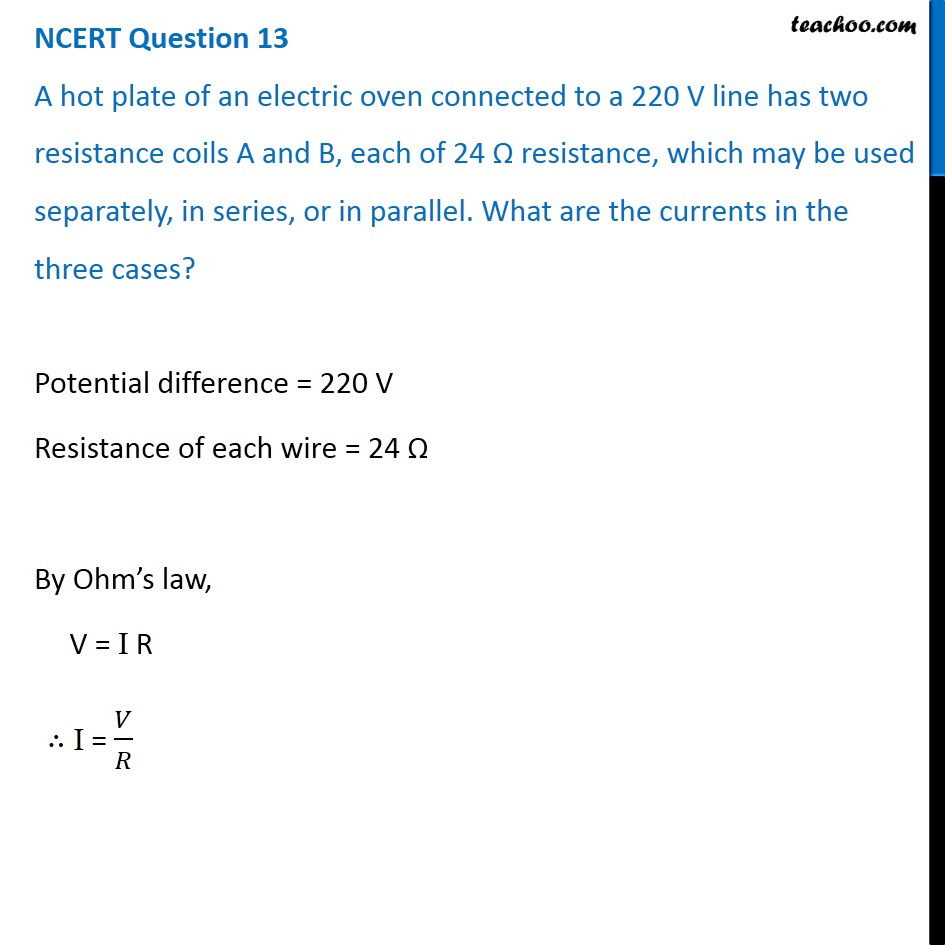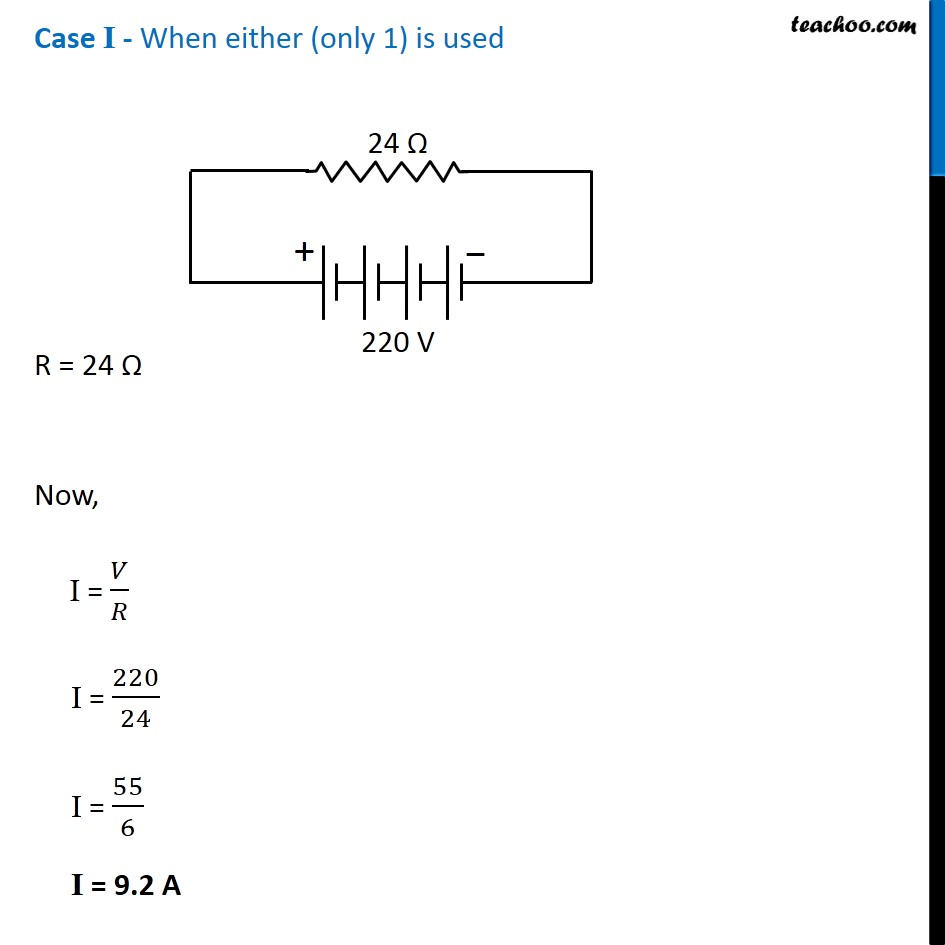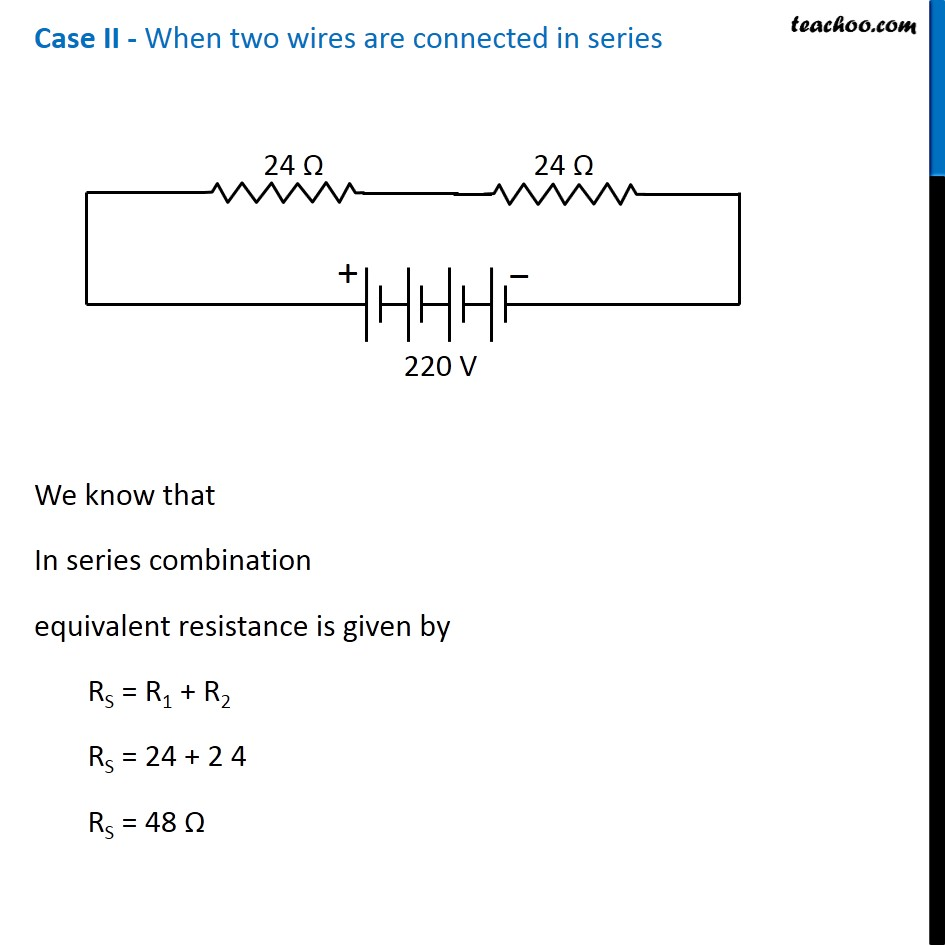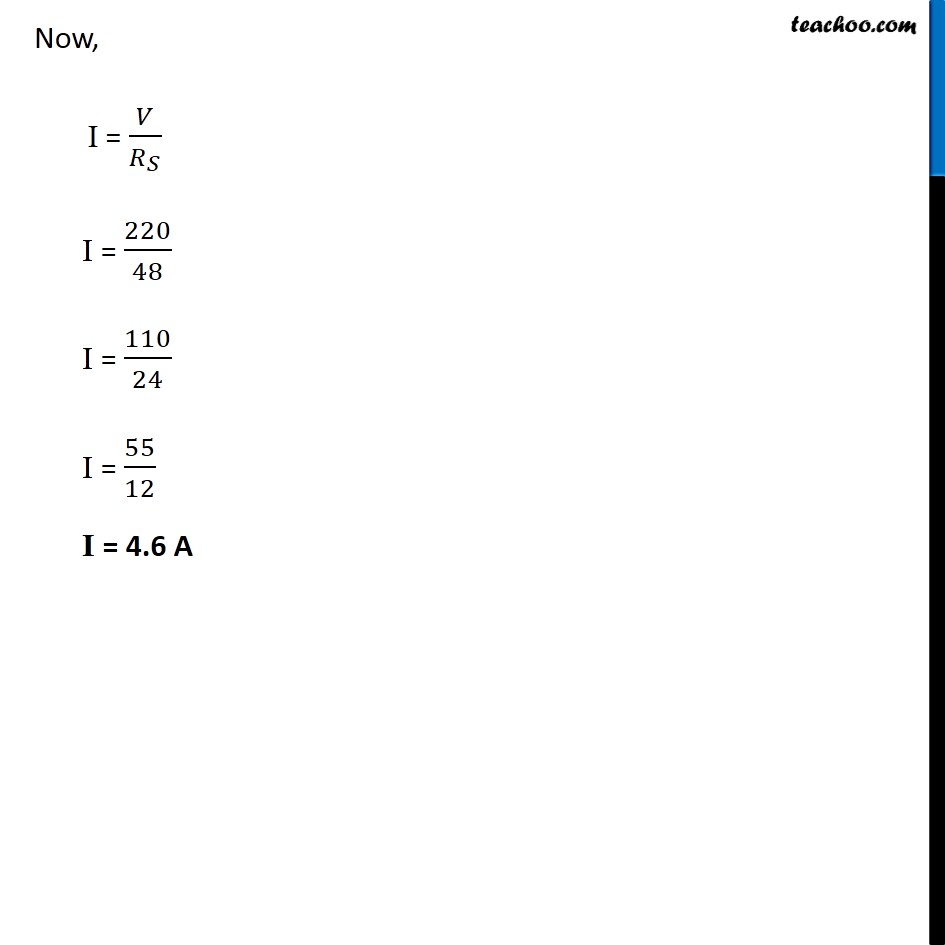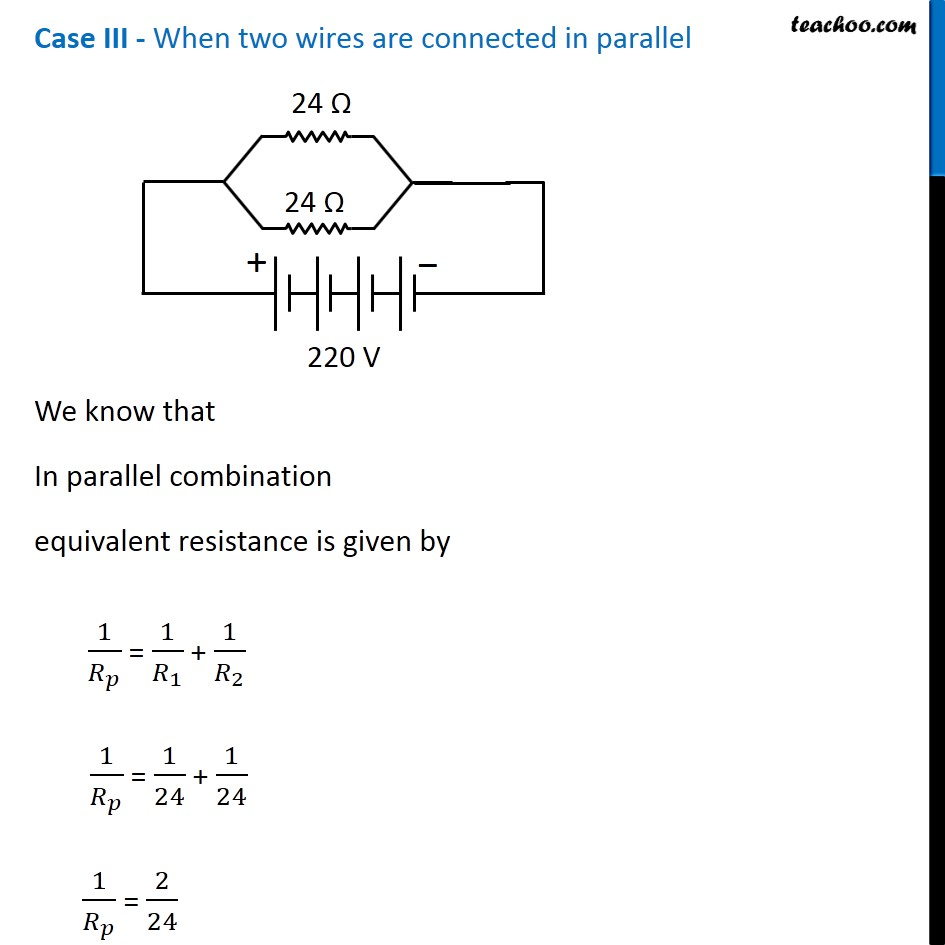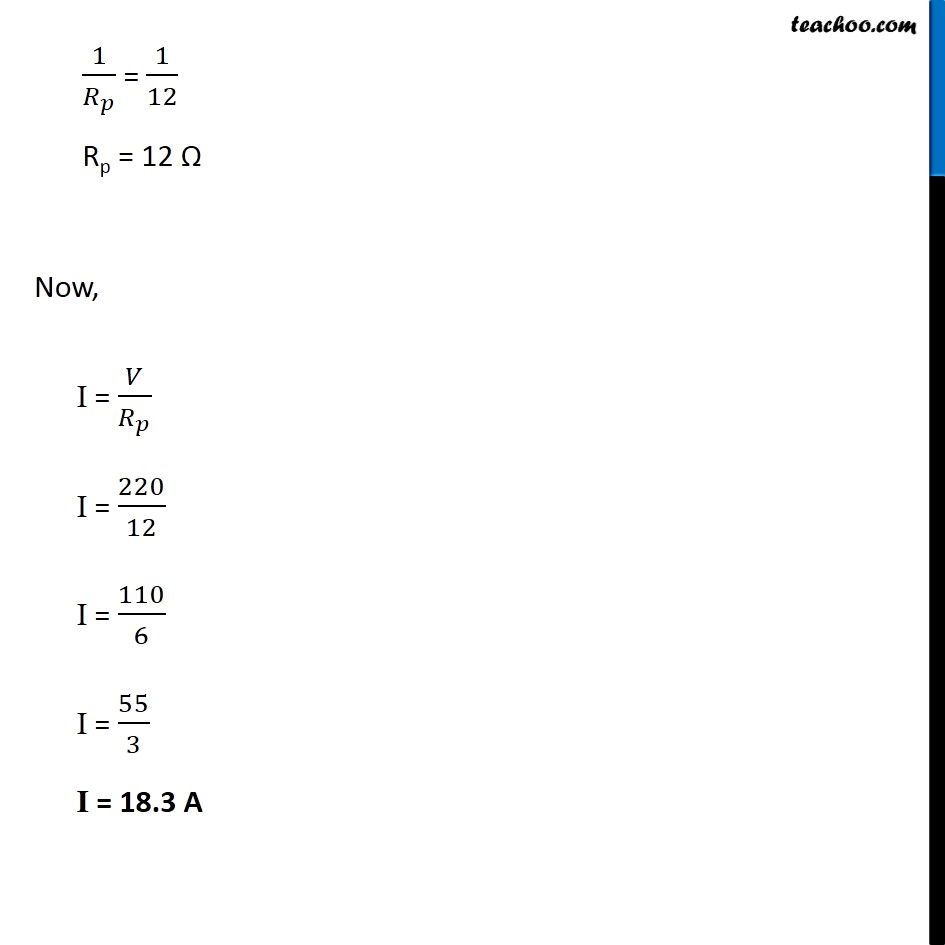Learn in your speed, with individual attention - Teachoo Maths 1-on-1 Class

### Transcript

NCERT Question 13 A hot plate of an electric oven connected to a 220 V line has two resistance coils A and B, each of 24 Ω resistance, which may be used separately, in series, or in parallel. What are the currents in the three cases? Potential difference = 220 V Resistance of each wire = 24 Ω By Ohm’s law, V = I R ∴ I = 𝑉/𝑅 Case I - When either (only 1) is used R = 24 Ω Now, I = 𝑉/𝑅 I = 220/24 I = 55/6 I = 9.2 A Case II - When two wires are connected in series We know that In series combination equivalent resistance is given by RS = R1 + R2 RS = 24 + 2 4 RS = 48 Ω Now, I = 𝑉/𝑅_𝑆 I = 220/48 I = 110/24 I = 55/12 I = 4.6 A Case III - When two wires are connected in parallel We know that In parallel combination equivalent resistance is given by 1/𝑅_𝑝 = 1/𝑅_1 + 1/𝑅_2 1/𝑅_𝑝 = 1/24 + 1/24 1/𝑅_𝑝 = 2/24 1/𝑅_𝑝 = 1/12 Rp = 12 Ω Now, I = 𝑉/𝑅_𝑝 I = 220/12 I = 110/6 I = 55/3 I = 18.3 A